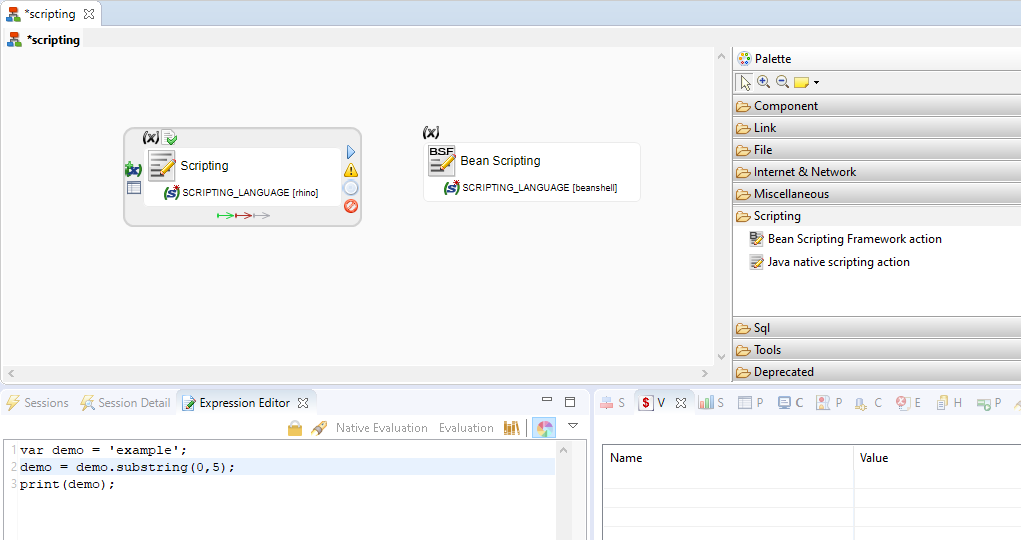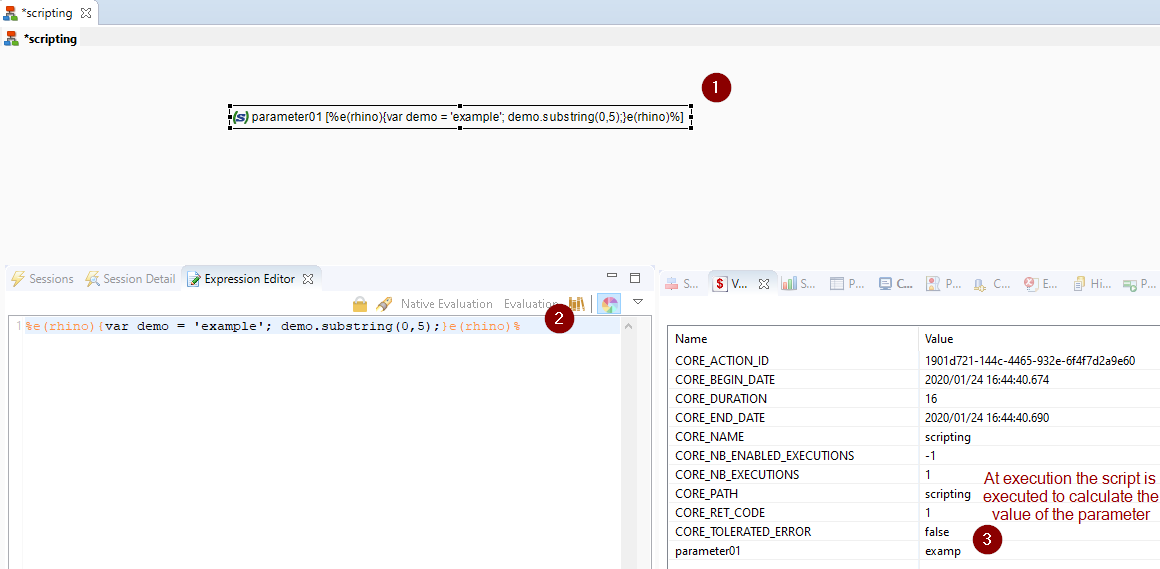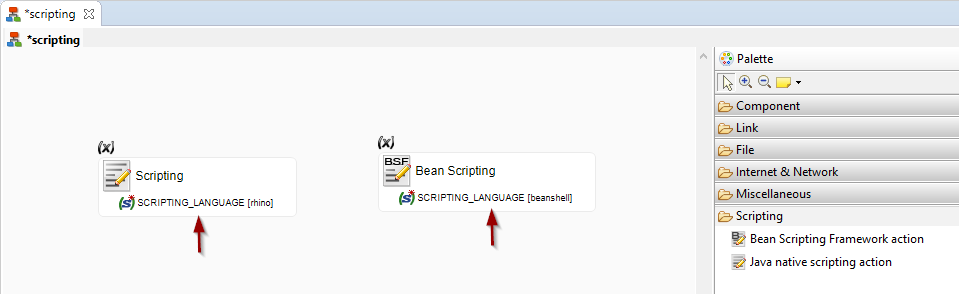# Use Scripting in Processes

Scripting allows you to customize the behavior of your processes. Using this feature, you can develop your own scripts and use them in Semarchy xDI processes.

## Use Scripting

Scripting is mainly used in scripting actions, and to configure text fields in processes and mappings.

### Scripting Actions

The first method to use scripting is with scripting actions (Bean Scripting Framework or Java Native Scripting) added to a process.

The following scripting languages are supported:

• Java Native Scripting action

• Rhino (Javascript)

• Nashorn

• Jython

• Groovy

• Bean Scripting Framework action

• Beanshell (Java)

• Javascript

• Jython

• Groovy

The action code is the script that you want to run.### Text Fields

Another method to use scripting is in properties that you define in Semarchy xDI Designer. For example, you can configure conditions, texts, process parameters, etc. with scripting.

 Scripting is used to define complex generation and execution conditions to control a process execution flow.

The syntax to use scripting in text fields is:

`%e(<language>){<script>}e(<language>)%`

Text fields support the following scripting languages:

• Rhino (Javascript)

• Nashorn

• Jython

• Groovy

In this context, the script is interpreted and the result of the script execution (return code) replaces the script in the field.

In the below example, the value of a parameter is computed from a script.### Scripting Language

When using scripting in Scripting Actions, you define the script to be used on the Scripting Language Parameter of the action.When using scripting in Text Fields, you can define the language using the following syntax: `%e(<language>){…​}e(<language>)%`

For example:

• `%e(rhino){…​}e(rhino)%`

• `%e(nashorn){…​}e(rhino)%`

• `%e(jython){…​}e(jython)%`

• `%e(groovy){…​}e(groovy)%`

### External libraries

To use in your classes and additional libraries not shipped in the runtime, you must add them in a Module and use that Module on the scripting Process Action.

 Modules are only supported in scripting actions, and not for scripts in text fields.

### Use Session Variables

Semarchy xDI stores information about each action and process. This information is available in session variables.

These variables can be used in the code/text of an action, in its parameters, and its metadata.
They can also be used in scripts, for example in conditions.

The syntax to use a variable is `\${variable_path}\$` where variable_path is the path to the variable.

A variable is defined in an action or a process, which may be contained in another process. This organization is similar to a file system organization, with the main process being the root element. You can access any variable with its absolute path or relative path

For example, consider the following process:

```LOAD_DW (process)
-> WRITE_FILE (action)
...
...
...```
`The _LOAD_DW_ process contains a sub-process named _LOAD_DIMENSIONS_, which contains a sub-process _LOAD_TIME_ which itself includes an action called _WRITE_FILE_. The return code of this action may be accessed in the `CORE_RET_CODE` variable, accessed by its absolute path:`
`\${LOAD_DW/LOAD_DIMENSIONS/LOAD_TIME/WRITE_FILE/CORE_RET_CODE}\$`

You can use this variable from READ_FILE action in the LOAD_TIME sub-process, using the following relative path (READ_FILE and WRITE_FILE are siblings in LOAD_TIME):

`\${../WRITE_FILE/CORE_RET_CODE}\$`

To use the return code of the current action in the action itself, you can use the following syntax.

`\${./CORE_RET_CODE}\$`

If the name of the parent process name is unknown, for example when the process is used by reference, you can use the `~/` syntax to return the full path. For example:

`\${~/WRITE_FILE/CORE_RET_CODE}\$`

In the previous case, `~/` resolves to `LOAD_DW/LOAD_DIMENSION/LOAD_TIME/`

## Use Scripts in Conditions

One of the most common use cases for scripting is complex conditions:

A condition is a script that returns a Boolean value, and that can use session variables using the `\${<variable>}\$` syntax.

The default language used for the conditions is JavaScript (Rhino) and the interpreter adds `%e(rhino){…​}e(rhino)%` around the code in conditions.

Simple Condition

The following condition checks whether the number of executions (`CORE_NB_EXECUTION`) of the AUTOMATION step is equal to `1`

`\${./AUTOMATION/CORE_NB_EXECUTIONS}\$==1`
Complex Condition

When a script is more complex than a simple expression, the context variable `__ctx__.retvalue` can be used to return the Boolean value from the script to the condition, as shown in the example below.

``````%e(rhino){
myVarValue = '\${~/MYVARIABLE}\$'; (1)
if (myVarValue.substring(0,1).equals(`R')) {
__ctx__.retValue = 'true'; (2)
}
else {
__ctx__.retValue = 'false'; (2)
}
}e(rhino)%``````
 1 Retrieve the value of the `MYVARIABLE` session variable. 2 Return the value to the condition using `__ctx__.retvalue`.

## The Scripting Context

When a script is interpreted, an object is passed to this script to provide access to the Runtime Engine features. This Context object is accessed using the `__ctx___` alias.

This object provides a list of methods for manipulating variables and values as well as a return value used for code substitution and condition evaluation.

The following sections describe the various elements available with this object.

### Return Value

The scripting context provides the retValue (`__ctx__.retValue`) variable.

This string variable is used to:

• Return a Boolean value to the condition interpreter.

• Return a string that replaces the script with its result during code generation.

### Publish Variable Values

The following method publishes a session variable.

``````public void publishVariable(String name, String value) {}
public void publishVariable(String name, String value, String type) {}``````

This method takes the following parameters:

• name: Path of the variable.

• value: Value of the variable.

• type: Type of the variable. The default value is String. Possible values are:

• Float

• Integer

• Long

• Boolean

• String

Publish a variable value

The following sample publishes a string variable called INCREMENTAL_MODE with the ACTIVE value to the parent process of the current action.

``__ctx__.publishVariable("../INCREMENTAL_MODE","ACTIVE");``

### Get Aggregated Variable Values

The following methods return the aggregated value for a variable. This aggregate is either a Sum, Average, Count, Min, or Max. The aggregate is performed within a given path.

``````public String sumVariable(String name) {}
public String sumVariable(String name, String startingPath) {}
public String averageVariable(String name) {}
public String averageVariable(String name, String startingPath) {}
public String countVariable(String name) {}
public String countVariable(String name, String startingPath) {}
public String minVariable(String name) {}
public String minVariable(String name, String startingPath) {}
public String maxVariable(String name) {}
public String maxVariable(String name, String startingPath) {}``````

These methods take the following parameters:

• name: Name of the variable

• startingPath: Path into which the variable values are aggregated. If this parameter is omitted, the values are aggregated for the entire Session.

Aggregate variable values

The following example aggregates the numbers of rows processed for the LOAD_DIMENSION process and all its sub-processes and steps.

``nb_rows = __ctx__.sumVariable("SQL_NB_ROWS","../LOAD_DIMENSION");``

The following method returns the current bind iteration number for bind link. It takes no input parameter.

``public long getCurrentBindIteration() {}``

### Get Variable Values

The following method returns the value of a session variable

``public String getVariableValue(String path) {}``

This method takes the following parameter:

• path: Variable path.

Retrieve a variable value

The following sample retrieves the value of the `CORE_SESSION_ID` variable.

``session_id = __ctx__.getVariableValue("/CORE_SESSION_ID");``

### Get Variable Cumulative Value

When an action iterates due to a bind or a loop, the variables store their value for the latest iteration.

This method retrieves the cumulated values for numeric variables.

``public Object getVariableCumulativeValue(String name) {}``

This method takes the following parameters:

• Name: Path of the numeric variable.

### Get Variable Tree

This method returns a `treeMap` object containing the variables corresponding to certain criteria.

``````public Map<String, IVariable> getVariableTreeByName(String name) {}
public Map<String, IVariable> getVariableTreeByName(String name, String startingPath) {}
public Map<String, IVariable> getVariableTreeByName(String name, boolean withErrors) {}
public Map<String, IVariable> getVariableTreeByName(String name, String startingPath, boolean withErrors)``````

It takes the following parameters:

• name: Name of the published variable.

• startingPath: Path from which the variable must be searched (The default value is `~/`)

• withErrors: Boolean value. If set to `true`, only the variables from steps in error are retrieved. It is set to `false` by default.

The returned Java Map object has the name of the action as the key and its value is a variable object with the following methods.

``````public Object getCumulativeValue(); // variable cumulated value (numbers only)
public String getShortName();       // variable name.
public String getName();            // variable name with path.
public String getActionName();      // action name with path.
public String getType();            // variable type.
public String getValue();           // variable value.``````
Retrieve variables tree

The following sample retrieves the stack trace for all the steps in error.

Example 1. Retrieve stack traces (Groovy)
``````%e(groovy){
def a = ""
def tree = __ctx__.getVariableTreeByName("CORE_STACK_TRACE","~/",true)
if (tree.size() != 0) {
def es=tree.entrySet()
es.each{
a = a+ "-- ACTION --> " + it.key + "\n"
a = a+ it.value.getValue() +"\n\n"
}
__ctx__.retValue = a
}
}e(groovy)%``````
Example 2. Retrieve stack traces (Javascript)
``````%e(rhino){
importPackage(java.util);
a = "";
tree = __ctx__.getVariableTreeByName("CORE_STACK_TRACE","~/",true);
if (tree.size() != 0) {
for (i= tree.keySet().iterator() ; i.hasNext() ; ){
action = i.next();
maVar = tree.get(action);
a = a+ "-- ACTION --> " + action + "\n";
a = a+ maVar.getValue() +"\n\n";
}
__ctx__.retValue = a
}
}e(rhino)%``````

### Get Variable List

This method works like getVariableTreeByName but returns a list of variables instead of a Java Map.

``````public List<IVariable> getLstVariablesByName(String name) {}
public List<IVariable> getLstVariablesByName(String name, boolean withErrors) {}
public List<IVariable> getLstVariablesByName(String name, String startingPath) {}
public List<IVariable> getLstVariablesByName(String name, String startingPath, boolean withErrors) {}``````
Retrieve variables list
Example 3. Retrieve stack traces (Groovy)
``````%e(groovy){
def a = ""
def lst = __ctx__.getLstVariablesByName("V1","~/")
if (lst.size() != 0) {
for (var in lst) {
a =a + var.getValue() + "\n"
}
__ctx__.retValue = a
}
}e(groovy)%``````
Example 4. Retrieve stack traces (Javascript)
``````%e(rhino){
importPackage(java.util);
a = "";
lst = __ctx__.getLstVariablesByName("V1","~/");
if (lst.size() != 0) {
for (i=0;i<lst.size();i++){
a = a+ "-- Value --> " + lst.get(i).getValue() +"\n";
}
__ctx__.retValue = a;
}
}e(rhino)%``````

### Create Bind Prepared Statement

This method returns an object allowing to produce a custom set of Bind columns in Scripting, which can then be used through an outgoing Bind link.

``public PreparedStatement createBindedPreparedStatement() {}``

This object allows manipulating the column definition as well as publishing rows.

#### Manage Column Definition

The following methods can be used to define the properties of a column.

``````public void setColumn(int columnId, String columnName);
public void setColumn(int columnId, String columnName, String dataType)
public void setColumn(int columnId, String columnName, String dataType, int precision);
public void setColumn(int columnId, String columnName, String dataType, int precision, int scale);``````

#### Update Column Properties

The following methods can be used to update the properties of a column.

``````public void setColumnName(int columnId, String columnName);
public void setColumnPrecision(int columnId, int precision);
public void setColumnType(int columnId, String dataType);``````

#### Set the Value

The following methods can be used to set or update the value of a column in the current row.

``````public void setBigDecimal(int columnId, BigDecimal value);
public void setBoolean(int columnId, boolean value);
public void setBytes(int columnId, byte[] value);
public void setDate(int columnId, Date value);
public void setDouble(int columnId, double value);
public void setInt(int columnId, int value);
public void setLong(int columnId, long value);
public void setString(int columnId, String value);
public void setTime(int columnId, Time value);
public void setTimestamp(int columnId, Timestamp value);``````

#### Publish a New Row

The following method can be used to publish a new row.

``public int executeUpdate()``
Example 5. Example: Create, execute a bind statement.
``````%e(rhino){
// Create the statement
ps=__ctx__.createBindedPreparedStatement();
// Definition of the columns
ps.setColumn(1,"TEST1"); // Set column 1
ps.setColumn(2,"TEST2","VARCHAR",255); // Set column 2
// First Bind Iteration
ps.setString(1,"VALUE1.1");
ps.setString(2,"VALUE2.1");
ps.executeUpdate();
// Second Bind Iteration
ps.setString(1,"VALUE3.1");
ps.setString(2,"VALUE3.2");
ps.executeUpdate();
}e(rhino)%``````
 Use this method in Scripting Actions to create your own Bind columns. This can be useful to iterate on a list of values for example in scripting and use the result as Bind values in the target action.

### Execute Commands

The following methods allow executing commands on runtimes:

• `executeCommand` runs a command on the current runtime.

• `executeCommands` runs a list of commands, separated by a defined separator character, on the current runtime.

• `executeRemoteCommand` runs a command on a remote runtime.

• `executeRemoteCommands` runs a list of commands, separated by a defined separator character, on a remote runtime.

``````public String executeCommand(String command) {}
public String executeCommands(String commands, String separator) {}
public String executeRemoteCommand(String host, int port, String command) {}
public String executeRemoteCommand(String host, int port, String command, User, encrypted Password) {}
public String executeRemoteCommands(String host, int port, String commands, String separator) {}
public String executeRemoteCommands(String host, int port, String commands, String separator, User, encrypted Password) {}``````

The available commands are the same as with the startCommand program.

These methods return the standard output produced by the command(s) execution.

This method takes the following parameters:

• command: Command executed by the runtime.

• commands: List of commands executed by the runtime, separated by the separator.

• separator: Separator used to separate the commands.

• host: Hostname or IP address of the remote runtime that will execute the commands, or a cluster name when the current runtime is configured in a runtime cluster.

• port: Port of the remote Runtime.

• user: User to authenticate to the runtime.

``````%e(rhino){